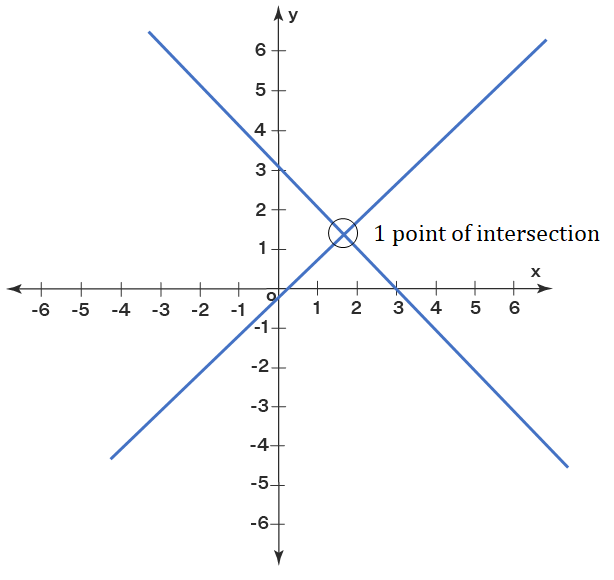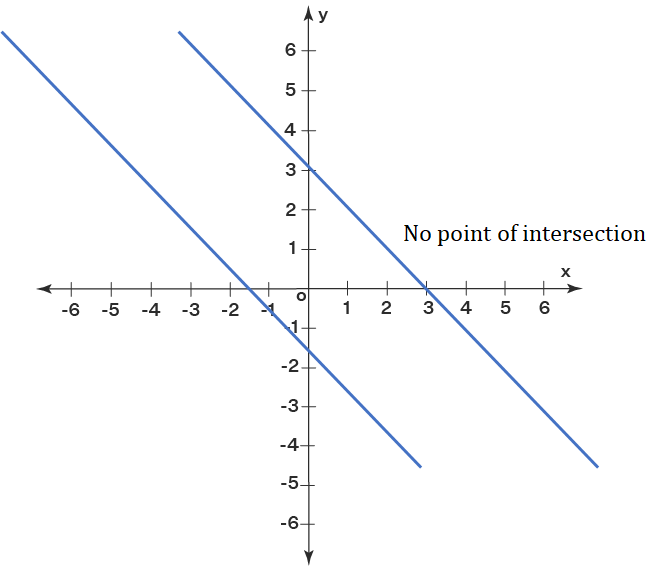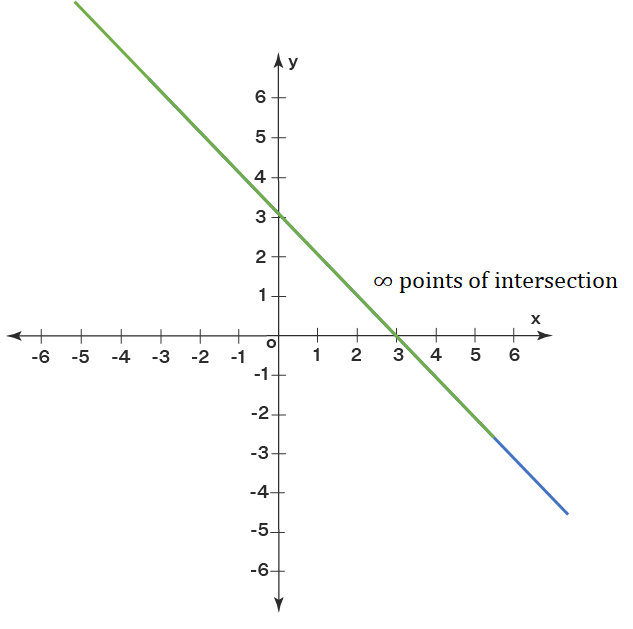# Concept: Simple Equations

INTRODUCTION

Most questions in Quantitative Aptitude ultimately boil down to solving equations. In this chapter we will look at simple or linear equations i.e., where the highest power of any variable is 1.

Solving a simultaneous set of linear equations means finding the values of variables which will satify the given set of equations.

Thumb Rule: We need as many independent equations as the number of variables in the given set of equations to find unique values of the given variables.

Example: If there are two variables, we need 2 independent equations to find a unique solution.

Most of the times you would come across 2 variable or 3 variable equations.

SINGLE VARIABLE EQUATIONS

A single variable linear equation is the easiest to solve. We bring all the variable terms on one side and all other terms on the other side.

Example: Solve for x: 3x + 6 = x + 18

Solution:
Given, 3x + 6 = x + 18

⇒ 3x - x = 18 - 6

⇒ 2x = 12

∴ x = 6

TWO VARIABLE EQUATIONS

A two variable equation is of the format 'ax + by + c = 0'. Here a is the coefficient of variable x and b is the coefficient of variable y.

SOLVING TWO VARIABLE EQUATIONS

SUBSTITUTION METHOD

In this method we substitute value of one of the variable from one equation in the other equation.

Example: Solve the following set of simultaneous equations:
13x + 2y = 36
11x + 3y = 37

Solution:
Given,
13x + 2y = 36 ...(1)
11x + 3y = 37 ...(2)

Step 1: Choose one of the equations and write one variable in terms of the other.
y = $\frac{\left(36 - 13x\right)}{2}$ ...(3)

Step 2: Substitute this value of y in other equation.
11x + 3 × $\frac{\left(36 - 13x\right)}{2}$ = 37
⇒ 22x + 3(36 - 13x) = 74
⇒ 22x + 108 - 39x = 74
⇒ 34 = 17x ⇒ x = 2

Step 3: Substitute value of y in (3)
y = (36 - 13 × 2)/2 = 5

∴ x = 2 and y = 5

ELIMINATION METHOD

In this method, we eliminate one of the variables.

Example: Solve the following set of simultaneous equations:
13x + 2y = 36
11x + 3y = 37

Solution:
Given,
13x + 2y = 36 ...(1)
11x + 3y = 37 ...(2)

Step 1: Choose one of the two variables to be eliminated and make the coefficient of that variable same in both equaitons.
Here, we will eliminated y, since the coefficients of y are smaller. ∴ (1) × 3, (2) × 3
⇒ 39x + 6y = 108 ...(3)
⇒ 22x + 6y = 74 ...(4)

Now, (3) = (4), we get
⇒ 17x = 34
⇒ x = 2

Step 2: Substitute this value of x in any of the original two equations.
From (1) we get,
⇒ 13 × 2 + 2y = 36
⇒ 26 + 2y = 36
⇒ 2y = 10 ⇒ y = 5

Step 3: Substitute value of y in (3)
y = (36 - 13 × 2)/2 = 5

∴ x = 2 and y = 5

GRAPHICAL REPRESENTATION OF TWO VARIABLE EQUATIONS

Remember:
• A two variable equation ax + by + c = 0 represents a straight line on the x-y graph.
• Solution of 2 two variable equations is same as the intersection point of the lines representing the 2 equations.

Example: x + y = 1 and x - y = 1.
Solution for these 2 equations is x = 1 and y = 0.

Now let us plot the two equations.

Equation (1): x + y = 1 ⇒ y = 1 - x.Equation (2): x - y = 1 ⇒ y = x - 1.From the above graph we see that, a two variable equations represents a straight line and the solution for the given equations is same as the intersection point of the lines representing the equations.

NUMBER OF INTERSECTION POINTS OF TWO LINES

For two lines

a1x + b1y + c1 = 0 and
a2x + b2y + c2 = 0

There are 3 possiblities for number of points of intersections.

UNIQUE SOLUTION (INTERSECTING LINES)

The two given set of equations will have a unique solution if:

$\frac{{\mathrm{a}}_{1}}{{\mathrm{a}}_{2}}$  ≠  $\frac{{\mathrm{b}}_{1}}{{\mathrm{b}}_{2}}$NO SOLUTION (PARALLEL LINES)

The two given set of equations will have no solution if:

$\frac{{\mathrm{a}}_{1}}{{\mathrm{a}}_{2}}$ = $\frac{{\mathrm{b}}_{1}}{{\mathrm{b}}_{2}}$ ≠ $\frac{{\mathrm{c}}_{1}}{{\mathrm{c}}_{2}}$INFINITE SOLUTIONS (OVERLAPPING LINES)

The two given set of equations will have infinite solutions if:

$\frac{{\mathrm{a}}_{1}}{{\mathrm{a}}_{2}}$ = $\frac{{\mathrm{b}}_{1}}{{\mathrm{b}}_{2}}$ = $\frac{{\mathrm{c}}_{1}}{{\mathrm{c}}_{2}}$THREE VARIABLE EQUATIONS

SOLVING THREE VARIABLE EQUATIONS

Three vaiable equations can also be solved using the methods discussed above i.e., either substitution method or elimination method. Method to be used depends upon the problem.

Example: Solve the following set of simultaneous equations:
x + 2y + 3z = 14
2x + 3y + z = 11
3x + y + 2z = 11

Solution:
Given
x + 2y + 3z = 14 ...(1)
2x + 3y + z = 11 ...(2)
3x + y + 2z = 11 ...(3)

Here, we will use the elimination method.

Step 1: Choose two of the three equations and eliminate one of the variables.
[Note: It is best to choose the variable with smallest coefficients.]

Here, we choose equations (1) and (2) and eliminate x.

∴ (1) × 2 - (2)
⇒ (2x + 4y + 6z) - (2x + 3y + z) = 28 - 11
⇒ y + 5z = 17 ...(4)

Step 2: Choose two other equations and eliminate the same variable as in Step 1.

Here, we choose equations (1) and (3) and eliminate x.

∴ (1) × 3 - (3)
⇒ (3x + 6y + 9z) - (3x + y + 2z) = 42 - 11
⇒ 5y + 7z = 31 ...(5)

Now we have two new equations (4) and (5). These are two equations in two variable which we learnt how to solve previously.

Solving (4) and (5), we get
y = 2 and z = 3

Substituting these values of y and z in any of the original three equations we get x = 1.

∴ x = 1, y = 2 and z = 3

Example: 600 is divided amongst A, B and C such that A gets one-fifth of what B and C get together. B gets half of what A and C get together. Find the amount with each of the three people.

Solution:
Given,
A + B + C = 600 ...(1)

Here, we will use the substitution method.

A gets one-fifth of what B and C get together,
∴ A = ⅕(B + C)
⇒ B + C = 5A ...(2)

Substituting (2) in (1), we get
A + 5A = 600
⇒ A = 100 ...(3)

B gets half of what A and C get together,
∴ B = ½(A + C)
⇒ A + C = 2A ...(4)

Substituting (4) in (1), we get
B + 2B = 600
⇒ B = 200 ...(5)

Now substituting (3) and (5) in (1), we get
100 + 200 + C = 600
⇒ C = 300

∴ A = Rs. 100, B = Rs. 200 and C = Rs. 300

SPECIAL CASES

SPECIAL CASE 1: L & M METHOD

Here, we would be given 2 equations but three variables and we would have to find some combination of the three variables.

Example: Find 5x + 3y + 7z if,
4x + 2y + 5z = 30
6x + 4y + 9z = 50.

Solution:
Here we can observe that if we add the two equations and then divided by 2, we get the desired relations i.e., 5x + 3y + 7z

∴ 5x + 3y + 7z = (30 + 50)/2 = 40

Note: The problem may not be so easy always. We can use l & m method to solve more complex problems.

Example: Find 10x + 8y + 17z if,
4x + 2y + 5z = 30
6x + 4y + 9z = 50.

Solution:
Given,
4x + 2y + 5z = 30 ...(1)
6x + 4y + 9z = 50 ...(2)

Here we multiply the first equation with l and second equation with m and then add them.

Equation (1): 4lx + 2ly + 5lz = 30l

Equation (2): 6mx + 4my + 9mz = 50m

(4l + 6m)x + (2l + 4m)y + (5l + 9m)z = 30l + 50m ...(3)

LHS should be same as 10x + 8y + 17z

⇒ Coefficient of x: 4l + 6m = 10
⇒ Coefficient of y: 2l + 4m = 8
⇒ Coefficient of z: 5l + 9m = 17

Solving the above three equations we get l = -2 and m = 3

⇒ 10x + 8y + 17z = 30 × -2 + 50 × 3 = 90

SPECIAL CASE 2: RATIO OF COEFFICIENTS OF TWO VARIABLES IS SAME

When 2 equations are given in three variables, value of one of the variables can be found if the ratio of coefficients of other two variables is same.

Example: Find the value of y
4x + 2y + 6z = 30
6x + 4y + 9z = 50.

Solution:
Given,
4x + 2y + 6z = 30 ...(1)
6x + 4y + 9z = 50 ...(2)

Since we want to find the value of y, we can eliminate either x or z. Let us eliminate x

(1) × 3 - (2) × 2

⇒ (12x + 6y + 18z) - (12x + 8y + 18) = 90 - 100
⇒ -2y = -10
∴ y = 5

Although we had only equations but three variables, we could find a unique value of one of the variables, since the ratio of coefficients of other two variables is same.

If we tried to eliminate z in the previous example we would get the same result, i.e., y = 5

Note: x and z will have infinite possible value. For all those infinite possible values, y will always be 5.

## Feedback

Help us build a Free and Comprehensive Preparation portal for various competitive exams by providing us your valuable feedback about Apti4All and how it can be improved.